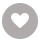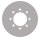# 一课一练.六年级数学.第一学期电子书租阅

71人正在读 | 0人评论6.2

• 读书简介
• 目录
• 累计评论(0条)
• 读书简介
• 目录
• 累计评论(0条)

Preface to the English Language Edition

Chinese-English Mathematics Glossary for Grade 6（First Semester）中英文数学词汇对照表（六年级第一学期）

Chapter 1 Divisibility of numbers

1.1 The meaning of integers and divisibility

I.Multiple choice questions

II.Fill in the blanks

III.Questions that require solutions

1.2 Factors and multiples

I.Multiple choice questions

II.Fill in the blanks

III.Questions that require solutions

1.3 Numbers divisible by 2 and 5

I.Multiple choice questions

II.Fill in the blanks

III.Questions that require solutions

1.4（1）Prime numbers，composite numbers and prime factorisation

I.Multiple choice questions

II.Fill in the blanks

III.Questions that require solutions

1.4（2）Prime numbers，composite numbers and prime factorisation

I.Multiple choice questions

II.Fill in the blanks

III.Questions that require solutions

1.5 Common factors and highest common factors

I.Multiple choice questions

II.Fill in the blanks

III.Questions that require solutions

1.6 Common multiples and lowest common multiples

I.Multiple choice questions

II.Fill in the blanks

III.Questions that require solutions

Unit test 1（Paper A）

I.Multiple choice questions

II.Fill in the blanks

III.Questions that require solutions

Unit test 1（Paper B）

I.Multiple choice questions

II.Fill in the blanks

III.Questions that require solutions

Chapter 2 Fractions

2.1 Fractions and division

I.Multiple choice questions

II.Fill in the blanks

III.Questions that require solutions

2.2（1）Basic properties of fractions

I.Multiple choice questions

II.Fill in the blanks

III.Questions that require solutions

2.2（2）Basic properties of fractions

I.Multiple choice questions

II.Fill in the blanks

III.Questions that require solutions

2.2（3）Basic properties of fractions

I.Multiple choice questions

II.Fill in the blanks（Fill in with a fraction in each of the questions in this section）

III.Questions that require solutions

2.3 Comparison of fractions

I.Multiple choice questions

II.Fill in the blanks

III.Questions that require solutions

2.4（1）Addition and subtraction of fractions

I.Fill in the blanks

II.Calculation questions

III.Questions that require solutions

2.4（2）Addition and subtraction of fractions

I.Multiple choice questions

II.Fill in the blanks

III.Questions that require solutions

2.4（3）Addition and subtraction of fractions

I.True or false.

II.Questions that require solutions

2.5 Multiplication of fractions

I.Multiple choice questions

II.Fill in the blanks

III.Questions that require solutions

2.6（1）Division of fractions

I.Multiple choice questions

II.Fill in the blanks

III.Questions that require solutions

2.6（2）Division of fractions

I.Multiple choice questions

II.Fill in the blanks

III.Questions that require solutions

2.7（1）Converting between fractions and decimals

I.Multiple choice questions

II.Fill in the blanks

III.Questions that require solutions

2.7（2）Converting between fractions and decimals

I.Multiple choice questions

II.Fill in the blanks

III.Questions that require solutions

2.8 Mixed four operations with fractions and decimals

I.Multiple choice questions

II.Questions that require solutions

2.9 Application of fraction operations

I.Fill in the blanks

II.Questions that require solutions

Unit test 2（Paper A）

I.Multiple choice questions

II.Fill in the blanks

III.Questions that require solutions

Unit test 2（Paper B）

I.Multiple choice questions

II.Fill in the blanks

III.Questions that require solutions

Chapter 3 Ratio and proportion

3.1 The meaning of ratio

I.Multiple choice questions

II.Fill in the blanks

III.Questions that require solutions

3.2 Basic properties of ratio

I.Multiple choice questions

II.Fill in the blanks

3.3 Proportion

I.Multiple choice questions

II.Fill in the blanks

3.4 The meaning of percentage

I.Multiple choice questions

II.Fill in the blanks

3.5（1）Application of percentages

I.Multiple choice questions

II.Fill in the blanks

3.5（2）Application of percentages

I.Multiple choice questions

II.Fill in the blanks

3.5（3）Application of percentages

I.Multiple choice questions

II.Fill in the blanks

3.5（4）Application of percentages

I.Multiple choice questions

II.Fill in the blanks

3.6 Equally likely events

I.Multiple choice questions

II.Fill in the blanks

Unit test 3（Paper A）

I.Multiple choice questions

II.Fill in the blanks

Unit test 3（Paper B）

I.Multiple choice questions

II.Fill in the blanks

Chapter 4 Circles and sectors

4.1 Circumferences of circles

I.Multiple choice questions

II.Fill in the blanks

II.Questions that require solutions

4.2 Arc length

I.Multiple choice questions

II.Fill in the blanks

III.Questions that require solutions

4.3（1）The area of a circle

I.Multiple choice questions

II.Fill in the blanks

III.Questions that require solutions

4.3（2）The area of a circle

I.Multiple choice questions

II.Fill in the blanks

III.Questions that require solutions

4.4（1）The area of a sector

I.Multiple choice questions

II.Fill in the blanks

III.Questions that require solutions

4.4（2）The area of a sector

I.Multiple choice questions

II.Fill in the blanks

III.Questions that require solutions

Unit test 4（Paper A）

I.Multiple choice questions

II.Fill in the blanks

III.Questions that require solutions

IV.Comprehensive questions

Unit test 4（Paper B）

I.Multiple choice questions

II.Fill in the blanks

III.Questions that require solutions

IV.Questions that require solutions

Mid-term test（Paper A）

Mid-term test（Paper B）

End of term test（Paper A）

End of term test（Paper B）

01

￥14.80
02

￥9.90
03

￥9.99
04

￥8.99
05

￥1.99
06

￥7.00
07

￥4.99
08

￥9.99
09

￥9.99
10

### 小学生作文快速入门与提高￥5.99

￥5.99
•关注我们

最受欢迎的阅读产品

关注我们：

•关于我们

欢迎反馈宝贵意见给我们

客服书吧：当当云阅读问答